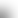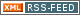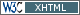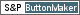www.LinuxHowtos.org howtos, tips&tricks and tutorials for linuxfrom small one page howto to huge articles all in one place

search text in:

Poll
Which kernel version do you use?

poll results

using iotop to find disk usage hogs

using iotop to find disk usage hogs

words:

887

views:

152935

userrating:

average rating: 1.8 (88 votes) (1=very good 6=terrible)

May 25th. 2007:
Words

486

Views

246162

Workaround and fixes for the current Core Dump Handling vulnerability affected kernels

Workaround and fixes for the current Core Dump Handling vulnerability affected kernels

words:

161

views:

129355

userrating:

average rating: 1.3 (28 votes) (1=very good 6=terrible)

April, 26th. 2006:

You are here: manpages

# dc

Section: User Commands (1)
Updated: 2008-05-22

## NAME

dc - an arbitrary precision calculator

## SYNOPSIS

dc [-V] [--version] [-h] [--help]
[-e scriptexpression] [--expression=scriptexpression]
[-f scriptfile] [--file=scriptfile]
[file ...]

## DESCRIPTION

dc is a reverse-polish desk calculator which supports unlimited precision arithmetic. It also allows you to define and call macros. Normally dc reads from the standard input; if any command arguments are given to it, they are filenames, and dc reads and executes the contents of the files before reading from standard input. All normal output is to standard output; all error output is to standard error.

A reverse-polish calculator stores numbers on a stack. Entering a number pushes it on the stack. Arithmetic operations pop arguments off the stack and push the results.

To enter a number in dc, type the digits (using upper case letters A through F as "digits" when working with input bases greater than ten), with an optional decimal point. Exponential notation is not supported. To enter a negative number, begin the number with ``_''. ``-'' cannot be used for this, as it is a binary operator for subtraction instead. To enter two numbers in succession, separate them with spaces or newlines. These have no meaning as commands.

## OPTIONS

dc may be invoked with the following command-line options:
-V
--version
Print out the version of dc that is being run and a copyright notice, then exit.
-h
--help
Print a usage message briefly summarizing these command-line options and the bug-reporting address, then exit.
-e script
--expression=script
Add the commands in script to the set of commands to be run while processing the input.
-f script-file
--file=script-file
Add the commands contained in the file script-file to the set of commands to be run while processing the input.

If any command-line parameters remain after processing the above, these parameters are interpreted as the names of input files to be processed. A file name of - refers to the standard input stream. The standard input will processed if no script files or expressions are specified.

## Printing Commands

p
Prints the value on the top of the stack, without altering the stack. A newline is printed after the value.
n
Prints the value on the top of the stack, popping it off, and does not print a newline after.
P
Pops off the value on top of the stack. If it it a string, it is simply printed without a trailing newline. Otherwise it is a number, and the integer portion of its absolute value is printed out as a "base (UCHAR_MAX+1)" byte stream. Assuming that (UCHAR_MAX+1) is 256 (as it is on most machines with 8-bit bytes), the sequence KSK0k1/_1Ss [ls*]Sxd0>x [256~Ssd0<x]dsxxsx[q]Sq[Lsd0>qaPlxx] dsxxsx0sqLqsxLxLK+k could also accomplish this function. (Much of the complexity of the above native-dc code is due to the ~ computing the characters backwards, and the desire to ensure that all registers wind up back in their original states.)
f
Prints the entire contents of the stack without altering anything. This is a good command to use if you are lost or want to figure out what the effect of some command has been.

## Arithmetic

+
Pops two values off the stack, adds them, and pushes the result. The precision of the result is determined only by the values of the arguments, and is enough to be exact.
-
Pops two values, subtracts the first one popped from the second one popped, and pushes the result.
*
Pops two values, multiplies them, and pushes the result. The number of fraction digits in the result depends on the current precision value and the number of fraction digits in the two arguments.
/
Pops two values, divides the second one popped from the first one popped, and pushes the result. The number of fraction digits is specified by the precision value.
%
Pops two values, computes the remainder of the division that the / command would do, and pushes that. The value computed is the same as that computed by the sequence Sd dld/ Ld*- .
~
Pops two values, divides the second one popped from the first one popped. The quotient is pushed first, and the remainder is pushed next. The number of fraction digits used in the division is specified by the precision value. (The sequence SdSn lnld/ LnLd% could also accomplish this function, with slightly different error checking.)
^
Pops two values and exponentiates, using the first value popped as the exponent and the second popped as the base. The fraction part of the exponent is ignored. The precision value specifies the number of fraction digits in the result.
|
Pops three values and computes a modular exponentiation. The first value popped is used as the reduction modulus; this value must be a non-zero number, and should be an integer. The second popped is used as the exponent; this value must be a non-negative number, and any fractional part of this exponent will be ignored. The third value popped is the base which gets exponentiated, which should be an integer. For small integers this is like the sequence Sm^Lm%, but, unlike ^, this command will work with arbitrarily large exponents.
v
Pops one value, computes its square root, and pushes that. The maximum of the precision value and the precision of the argument is used to determine the number of fraction digits in the result.

Most arithmetic operations are affected by the ``precision value'', which you can set with the k command. The default precision value is zero, which means that all arithmetic except for addition and subtraction produces integer results.

## Stack Control

c
Clears the stack, rendering it empty.
d
Duplicates the value on the top of the stack, pushing another copy of it. Thus, ``4d*p'' computes 4 squared and prints it.
r
Reverses the order of (swaps) the top two values on the stack. (This can also be accomplished with the sequence SaSbLaLb.)
R
Pops the top-of-stack as an integer n. Cyclically rotates the top n items on the updated stack. If n is positive, then the rotation direction will make the topmost element the second-from top; if n is negative, then the rotation will make the topmost element the n-th element from the top. If the stack depth is less than n, then the entire stack is rotated (in the appropriate direction), without any error being reported.

## Registers

dc provides at least 256 memory registers, each named by a single character. You can store a number or a string in a register and retrieve it later.

sr
Pop the value off the top of the stack and store it into register r.
lr
Copy the value in register r and push it onto the stack. The value 0 is retrieved if the register is uninitialized. This does not alter the contents of r.

Each register also contains its own stack. The current register value is the top of the register's stack.

Sr
Pop the value off the top of the (main) stack and push it onto the stack of register r. The previous value of the register becomes inaccessible.
Lr
Pop the value off the top of register r's stack and push it onto the main stack. The previous value in register r's stack, if any, is now accessible via the lr command.

## Parameters

dc has three parameters that control its operation: the precision, the input radix, and the output radix. The precision specifies the number of fraction digits to keep in the result of most arithmetic operations. The input radix controls the interpretation of numbers typed in; all numbers typed in use this radix. The output radix is used for printing numbers.

The input and output radices are separate parameters; you can make them unequal, which can be useful or confusing. The input radix must be between 2 and 16 inclusive. The output radix must be at least 2. The precision must be zero or greater. The precision is always measured in decimal digits, regardless of the current input or output radix.

i
Pops the value off the top of the stack and uses it to set the input radix.
o
Pops the value off the top of the stack and uses it to set the output radix.
k
Pops the value off the top of the stack and uses it to set the precision.
I
Pushes the current input radix on the stack.
O
Pushes the current output radix on the stack.
K
Pushes the current precision on the stack.

## Strings

dc has a limited ability to operate on strings as well as on numbers; the only things you can do with strings are print them and execute them as macros (which means that the contents of the string are processed as dc commands). All registers and the stack can hold strings, and dc always knows whether any given object is a string or a number. Some commands such as arithmetic operations demand numbers as arguments and print errors if given strings. Other commands can accept either a number or a string; for example, the p command can accept either and prints the object according to its type.

[characters]
Makes a string containing characters (contained between balanced [ and ] characters), and pushes it on the stack. For example, [foo]P prints the characters foo (with no newline).
a
The top-of-stack is popped. If it was a number, then the low-order byte of this number is converted into a string and pushed onto the stack. Otherwise the top-of-stack was a string, and the first character of that string is pushed back.
x
Pops a value off the stack and executes it as a macro. Normally it should be a string; if it is a number, it is simply pushed back onto the stack. For example, [1p]x executes the macro 1p which pushes 1 on the stack and prints 1 on a separate line.

Macros are most often stored in registers; [1p]sa stores a macro to print 1 into register a, and lax invokes this macro.

>r
Pops two values off the stack and compares them assuming they are numbers, executing the contents of register r as a macro if the original top-of-stack is greater. Thus, 1 2>a will invoke register a's contents and 2 1>a will not.
!>r
Similar but invokes the macro if the original top-of-stack is not greater than (less than or equal to) what was the second-to-top.
<r
Similar but invokes the macro if the original top-of-stack is less.
!<r
Similar but invokes the macro if the original top-of-stack is not less than (greater than or equal to) what was the second-to-top.
=r
Similar but invokes the macro if the two numbers popped are equal.
!=r
Similar but invokes the macro if the two numbers popped are not equal.
?
Reads a line from the terminal and executes it. This command allows a macro to request input from the user.
q
exits from a macro and also from the macro which invoked it. If called from the top level, or from a macro which was called directly from the top level, the q command will cause dc to exit.
Q
Pops a value off the stack and uses it as a count of levels of macro execution to be exited. Thus, 3Q exits three levels. The Q command will never cause dc to exit.

## Status Inquiry

Z
Pops a value off the stack, calculates the number of decimal digits it has (or number of characters, if it is a string) and pushes that number. The digit count for a number does not include any leading zeros, even if those appear to the right of the radix point.
X
Pops a value off the stack, calculates the number of fraction digits it has, and pushes that number. For a string, the value pushed is 0.
z
Pushes the current stack depth: the number of objects on the stack before the execution of the z command.

## Miscellaneous

!
Will run the rest of the line as a system command. Note that parsing of the !<, !=, and !> commands take precedence, so if you want to run a command starting with <, =, or > you will need to add a space after the !.
#
Will interpret the rest of the line as a comment.
:r
Will pop the top two values off of the stack. The old second-to-top value will be stored in the array r, indexed by the old top-of-stack value.
;r
Pops the top-of-stack and uses it as an index into the array r. The selected value is then pushed onto the stack. Note that each stacked instance of a register has its own array associated with it. Thus 1 0:a 0Sa 2 0:a La 0;ap will print 1, because the 2 was stored in an instance of 0:a that was later popped.

## BUGS

Email bug reports to bug-dc@gnu.org.

## Index

NAME
SYNOPSIS
DESCRIPTION
OPTIONS
Printing Commands
Arithmetic
Stack Control
Registers
Parameters
Strings
Status Inquiry
Miscellaneous
BUGS||- Powered by- Running on-
Copyright 2004-2020 Sascha Nitsch Unternehmensberatung GmbH::- Level Triple-A Conformance to Web Content Accessibility Guidelines 1.0 -
- Copyright and legal notices -